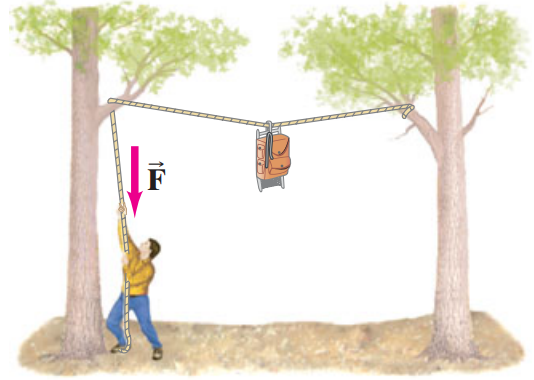Raúl's web!

# Problem 8 (easy problem)

The two trees are (l)m distance apart. A backpacker is trying to lift his pack out of the reach of bears. Calculate the magnitude of the force (FT)newtons that he must exert downward to hold a (m)kg backpack so that the rope sags at its midpoint by (y)m distance.

a)Please enter the following data to calculate the previous equation:

 Enter the distance so the ropes sags at its midpoint (y). Enter the distance between trees (l). Equation 1 result is (radians) Comment:

Please enter the following data to calculate the previous equation:

 Enter mass Enter the radians from the last equation Equation 2 result is Comment: